#### You may also likeThis comes in two parts, with the first being less fiendish than the second. Itâ€™s great for practising both quadratics and laws of indices, and you can get a lot from making sure that you find all the solutions. For a real challenge (requiring a bit more knowledge), you could consider finding the complex solutions.### Discriminating

You're invited to decide whether statements about the number of solutions of a quadratic equation are always, sometimes or never true.This will encourage you to think about whether all quadratics can be factorised and to develop a better understanding of the effect that changing the coefficients has on the factorised form.

# Approaching Asymptotes

##### Age 16 to 18This resource is from Underground Mathematics.

### Warm up

This is a graph of the function $f(x)=\dfrac{1}{x}.$The graph has two asymptotes.

How would you describe what an asymptote is?

## Main problem

Here are some descriptions or statements about asymptotes.

1. "An asymptote is a line which a curve gets closer and closer to but doesn't meet."
2. "An asymptote is a line which a curve approaches as $x$ tends to infinity."
3. "A curve can't cross an asymptote."
4. "Asymptotes are parallel to the coordinate axes."
5. "A graph can only have one asymptote parallel to each axis."
6. "Asymptotes occur when a function isn't defined for certain input values."
7. "A function tends to positive infinity on one side of an asymptote and tends to negative infinity on the other side."

Here are some examples of curves, some of which have asymptotes.  Use these to help you decide whether you agree with the statements above. Printable versions of these cards can be downloaded here

A                                                                             B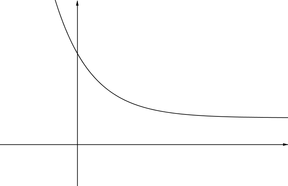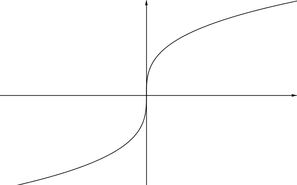C                                                                             D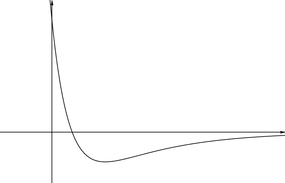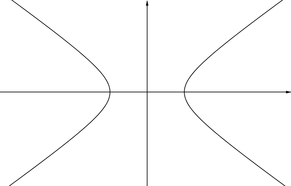E                                                                              F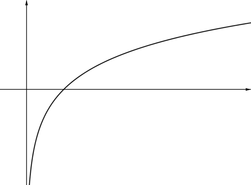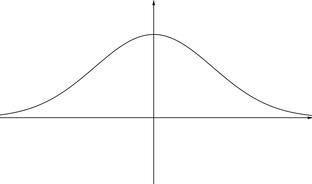G                                                                             H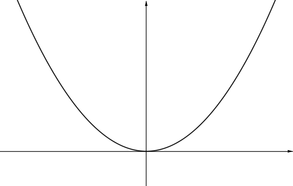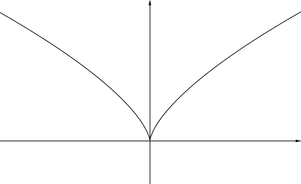I                                                                              J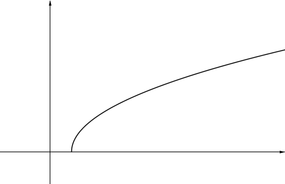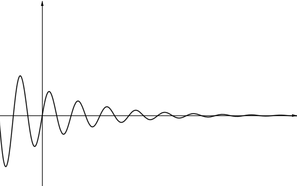K                                                                            L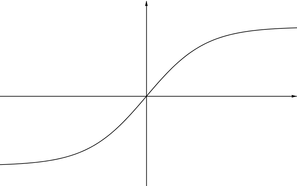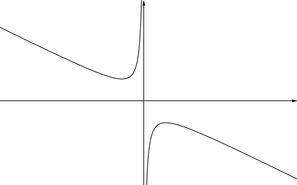M                                                                            N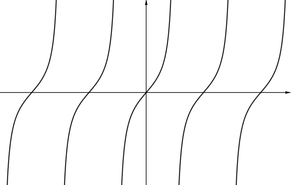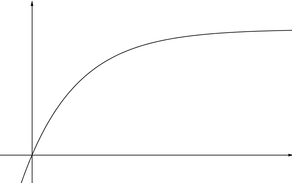O                                                                            P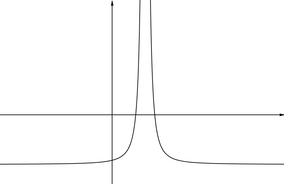This is an Underground Mathematics resource.

Underground Mathematics is hosted by Cambridge Mathematics. The project was originally funded by a grant from the UK Department for Education to provide free web-based resources that support the teaching and learning of post-16 mathematics.

Visit the site at undergroundmathematics.org to find more resources, which also offer suggestions, solutions and teacher notes to help with their use in the classroom.